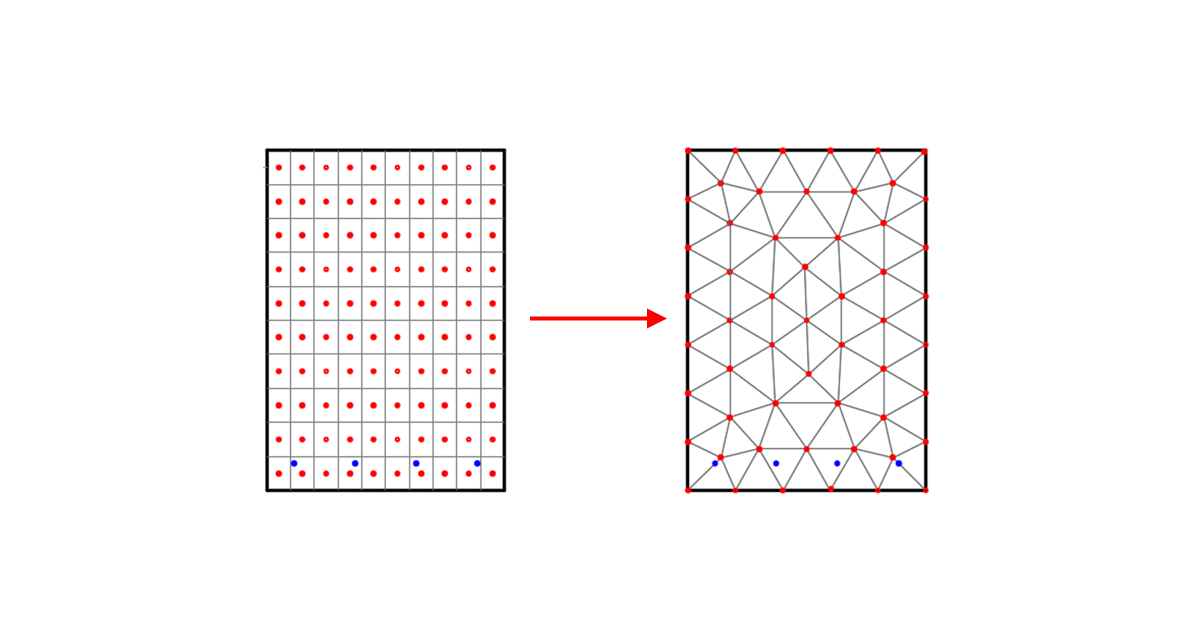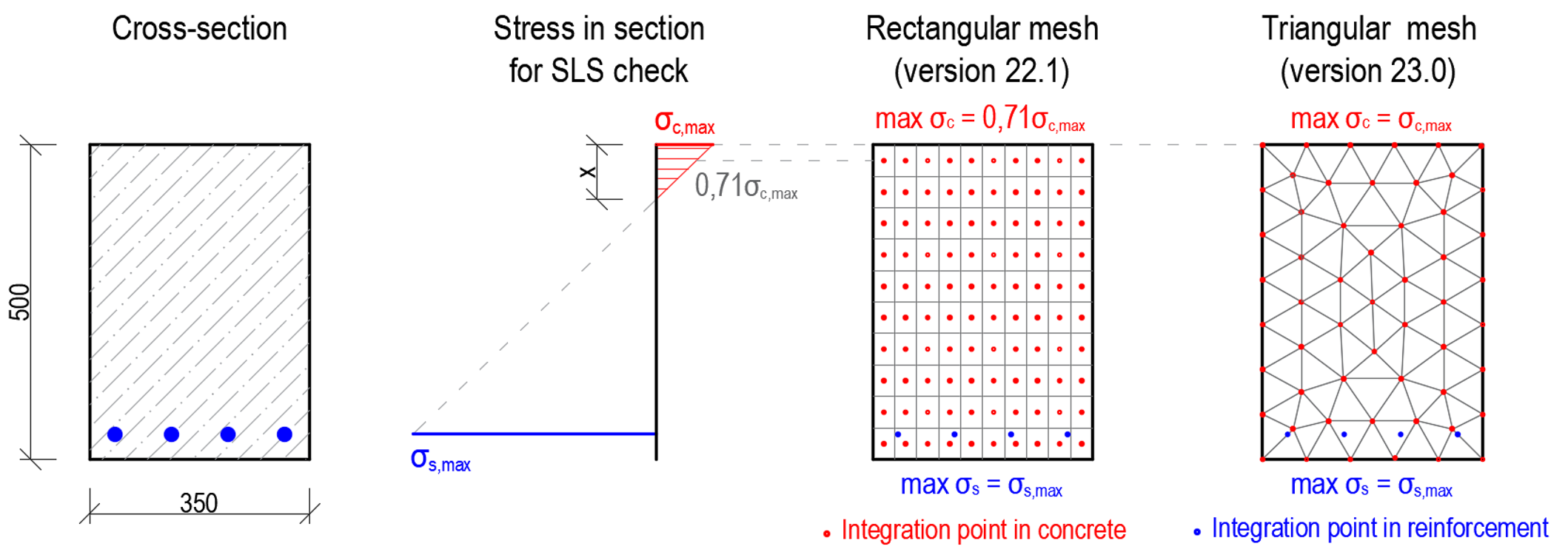### Choose language# Triangular mesh in concrete Member

$$A triangular mesh for cross-sections has been implemented in the Member application for concrete members. The rectangular mesh that was used previously was sufficient for members in bending only if the very fine mesh was defined. The reason is that the results are calculated in the integration points located in the center of gravity of each finite element, so we don't calculate the actual stress at the edge of the cross-section. Results are then very sensitive to mesh size, especially for members in bending, for which the compressive zone in concrete could be just a few centimeters high. Hence, many finite elements cause a slowing down of the calculation as well as the presentation of the results in the 3D scene.All these problems have been solved by implementing the triangular mesh for sections calculated by the MNA and GMNIA analysis. Integration points are located in nodes (finite element vertexes), so we get results at the edge of the cross-section to capture stress peaks in concrete due to bending. This brings many advantages to users. The results are not sensitive to mesh size, so by default, the mesh is rough (in comparison with rectangular mesh), leading to decreasing the calculation time and faster application response when working with results along with more precise and safer results.Available in the Enhanced edition of IDEA StatiCa Concrete Download the new version of IDEA StatiCa and try all the features Download new version$$